## Articulo

•Citado por SciELO
•Accesos

•Similares en SciELO

## versión impresa ISSN 1405-3322

### Bol. Soc. Geol. Mex vol.68 no.3 México dic. 2016

Artículos regulares

Neural networks for defining spatial variation of rock properties in sparsely instrumented media

1Geotecnia, Instituto de Ingeniería UNAM, Circuito Escolar s/n, Ciudad Universitaria, Delegación Coyoacán, Ciudad de México, C.P. 04510.

2División de Inyecciones y Mecánica de Rocas, Comisión Federal de Electricidad CFE, Augusto Rodin No.265, Delegación Benito Juárez, Ciudad de México, C.P. 03820.

Abstract:

Reliable information of the three-dimensional distribution of rock mass properties improves the design of secure and cost-effective civil structures. In this paper, a recurrent neural network is presented as an alternative to predict the spatial variation of some index properties of rock in sparsely instrumented media. The neural technique, from statistical learning models, is used to approximate functions that can depend on a large number of inputs that are generally unknown. From a reasonably simple neuronal model of two inhomogeneous rock volumes, the limited measured information is extrapolated and the properties in the entire mass can be estimated. Comparisons between in situ explorations versus the 3D-neuronal definition confirm the potential of the proposed method for characterizing the properties of masses with inhomogeneous properties. Such a representation is useful for design of economic realistic numerical modelling of rock volumes, maximizing information while minimizing cost.

Keywords: spatial variation analysis; index properties of rock, artificial intelligence, back propagation, recurrent neural networks

Resumen:

La información confiable de la distribución tridimensional de las propiedades del macizo rocoso mejora el diseño de estructuras civiles seguras y rentables. En este trabajo, se presenta una red neuronal recurrente como una alternativa para predecir la variación espacial de algunas propiedades índice de roca en medios escasamente instrumentados. La técnica neuronal, que forma parte de los modelos de aprendizaje estadístico, se utiliza para aproximar funciones que pueden depender de un gran número de entradas y que generalmente son desconocidas. Con un modelo neuronal, razonablemente simple, de dos volúmenes de roca no homogéneos, se extrapola la escasa información levantada en campo y se estiman las propiedades en toda la masa. Las comparaciones entre la exploración in situ y la definición neuro-3D confirma el potencial del método propuesto para la caracterización de propiedades de las masas con propiedades no homogéneas. Esta representación es útil para el modelado numérico realista y económico de volúmenes de roca, maximizando la información mientras se reducen los costos.

Palabras clave: análisis de variación espacial; propiedades índice de rocas, inteligencia artificial, retro-propagación, redes neuronales recurrentes

1. Introduction

In recent years, the assessment of the spatial variation of properties of rock masses has caught the attention of many researchers (e.g . Lashkaripour and Ghafoori, 2002; Ghobadi et al., 2005; Kocbay and Kilic, 2006; Ghafoori et al ., 2011; Uromeihy and Farrokhi, 2012). The relationship between rock-mass attributes and the distribution of potentially unstable areas, observed in the models, raises a concern. More refined modelling approaches are conducted on the basis of rock properties as design parameters to define crucial behaviours. Since the quality of prediction is affected by the accuracy of the data, there is demand for more fidelity when estimating 3D properties used in modelling.

In this paper, a new methodology for determining 3D properties of rock masses, a neural network (NN), particularly a recurrent neural network (RNN), is presented. NNs are trained with examples of the concepts they are trying to capture and internally organize themselves to be able to reconstruct the presented examples. NNs have the ability to produce correct, or nearly correct, responses when presented with partially incorrect or incomplete input data (stimuli ). They also are able to generalize rules from the cases on which they are trained and apply these rules to new stimuli . The main attributes of neural networks are their robustness to noise data and their ability to generalize to new input; in other words, a trained network is capable of providing sensible output when presented with input data that have not been used during training, even if these data contain random noise.

The geological and geotechnical analyses performed on a dam site (both abutments) are used to present the procedure for attaining a neuro-evaluation of some index properties of rock masses. The neural frame permits us to build on 3D displays of mass descriptors: rock quality designation (RQD), core recovery (%REC), number and inclination of fractures (#FRAC and °FRAC respectively) and material type (MT). Based on the information given in this paper, NNs are shown as able to predict with good approximation the spatial variation of rock properties. Because of their simplicity and demand of computational resources, NNs seems very useful particularly when extensive exploratory campaigns are prohibitive or not available, such as the dam example that is shown.

2. Basics of neural networks

The scope of this section is to make a brief induction to Artificial Neural Networks (or just Neural Networks NNs), much of the formality is skipped for the sake of simplicity. Detailed explanations and demonstrations can be found in Cybenko (1989), Hornik (1991), Hassoun (1995), Haykin (1999) and Csáji (2001).

Since the first neural model by McCulloch and Pitts (1943), hundreds of different models have been developed. Given that the function of NNs is to process information, they are used mainly in fields related to this topic. The wide variety of NNs used for engineering purposes works mainly in pattern recognition, forecasting, and data compression.

A NN is characterized by two main components: a set of nodes, and the connections between nodes. The nodes can be seen as computational units that receive external information (inputs) and process it to obtain an answer (output), this processing might be very simple (such as summing the inputs), or quite complex (a node might be another network itself). The connections (weights) determine the information flow between nodes. They can be unidirectional, when the information flows only in one sense, and bidirectional, when the information flows in either sense.

The interactions of nodes through the connections lead to a global behaviour of the network that is conceived as emergent "knowledge". Inspired by biological neurons (Figure 1), nodes, or artificial neurons, collect signals through connections as the synapses located on the dendrites or membrane of the organic neuron. When the signals received are strong enough (beyond a certain threshold) the neuron is activated and sends out a signal through the axon to another synapse and might activate other neurons. The higher the connections (weights) between neurons, the stronger the influence of the nodes connected on the modelled system.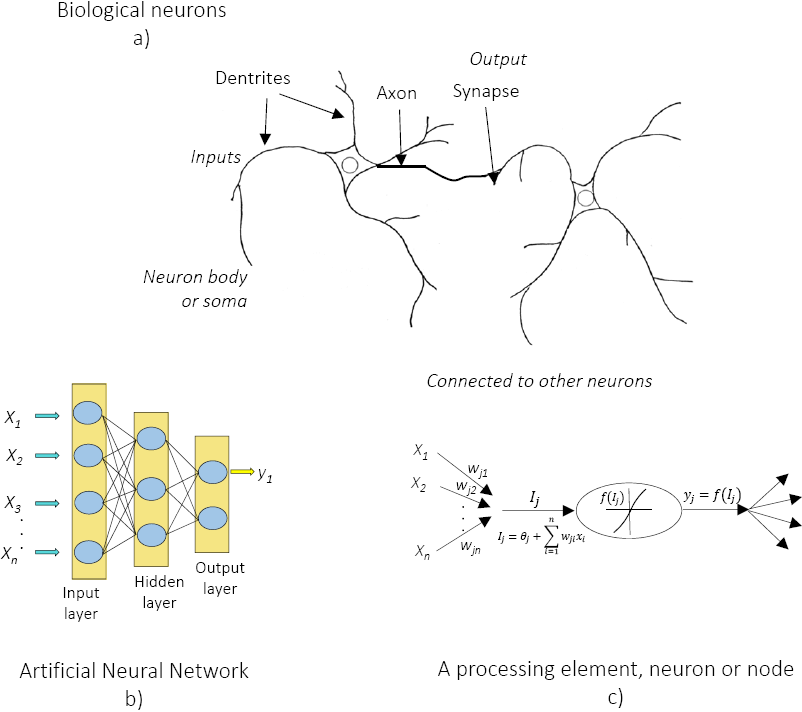Figure 1 Biological and artificial neural networks, xn are the inputs, wij are the weights, Ij is the identity matrix, θj is the bias, f(·) is the transfer function and yj is the network output.

By adjusting the weights the desired output of a NN for specific inputs can be obtained in a process that is known as learning or training. For NNs with hundreds or thousands of neurons, it would be quite complicated to find the required weights so it is necessary to use algorithms which can, massively, adjust the NN weights based on desired outputs. To become familiar with these NN concepts, see the exercise shown in Figure 2. In this example a layered network consisting of four artificial neurons is depicted (two neurons receive inputs and the other two present network outputs). The weights, assigned with each arrow, represent information flow. Consider that the neuron's activities are simply summing their inputs. Since the input neurons have only one input, their output will be the received input multiplied by a weight. The neurons on the output layer receive the numbers from both input neurons, multiply them by their respective weights and sum these quantities.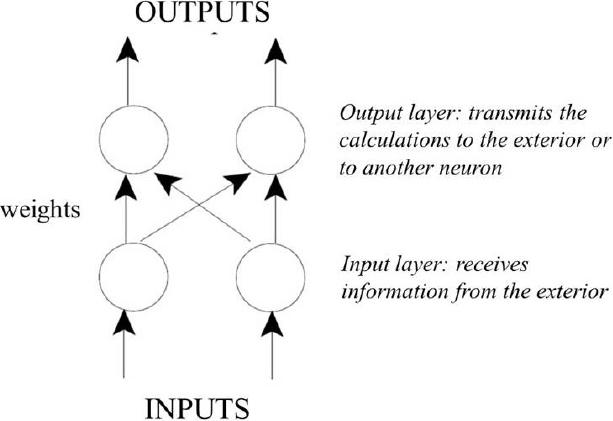Figure 2 A simple example of a NN: two inputs, two outputs, four weights.

Setting the weights equal to one means that the information will flow unaffected, but changing some critical weights the NN behaviour does dramatically. Perhaps it is not so complicated to adjust the weights of such a small network, its capabilities are quite limited. In a more skilled NN, hundreds or thousands of neurons will be necessary to execute the desired task and the methods to find the weights will be more elaborated. A scheme to discover weights by the training backpropagation algorithm (Rumelhart and McClelland, 1986), will be explained in the following subsection. It is one of the most commonly used method successful NN applications (Shahin et al., 2008; 2009; Moreshwar, 2013) and it is the one used in this investigation.

2.1. The Backpropagation Algorithm

The backpropagation algorithm (BP) (Rumelhart and McClelland, 1986) is used in layered feedforward NNs. This kind of networks is organized in layers that send their signals forward. The information is received from the exterior in the input layer, the network final calculation is given in an output layer, and the processing is developed in intermediate or hidden layers.

The BP algorithm uses supervised learning, which means that the network modeller provides the algorithm with examples of the inputs and their corresponding outputs (those that the network must approximate). The objective of the backpropagation algorithm is to reduce the difference between actual and expected results, and in doing so the NN is said to be "learning" from the data (examples or "training" records). The procedure begins with random weights and the goal is to adjust them so that the error will be minimal.

The activation function of the neurons in NN implementing the backpropagation algorithm is a weighted sum (the sum of the inputs xi multiplied by their respective weights wji ):

As can be seen, the neuron activation depends only on the inputs and the weights. If the output function would be the identity (activation = output) then the neuron would be called linear. But these have severe limitations, the most common output function is the sigmoidal function:

The sigmoidal function is very close to one for large positive numbers, 0.5 at zero, and very close to zero for large negative numbers. This allows a smooth transition between the low and high out-puts (close to zero or close to one). The goal of the training process is to obtain a desired output when certain inputs are given. Since the error is the difference between the actual and the desired output, the error depends on the weights, and we need to adjust the weights in order to minimize the error. In this investigation, the error function for the output of each neuron is defined as:

This error measure, always positive, is very convenient for the research purpose because it will be greater if the difference is big, and lesser if the difference is small. The error of the network will simply be the sum of the errors of all the neurons in the output layer:

In the BP algorithm once the output, inputs, and weights are known the weights adjustment is performed using the method of gradient descendent:

This formula can be interpreted as: the adjustment of each weight (Δwji ) will be the negative of a constant eta (η ) multiplied by the dependence of the previous weight on the error of the network, which is the derivative of E in respect to wji . The size of the adjustment will depend on O and on the contribution of the weight to the error of the function. That is, if the weight contributes a large amount to the error, the adjustment will be greater than if it contributes a smaller amount. Equation 5 is used until the appropriate weights are found and the error is minimal. Mathematical proof of the backpropagation algorithm can be found in Werbos (1994), and Jeremias et al . (2014).

Now it is necessary to find the derivative of E with respect to wji , which is the basic purpose of the BP algorithm. First, it is required to calculate how much the error depends on the output, which is the derivative of E with respect to Oj (from Equation 3).

and then, how much the output differs on the activation (from Equations 1 and 2):

It can be seen that (from Equations 6 and 7):

so the adjustment to each weight will be (from Equations 5 and 8):

Equation (9) can be used for training a NN with two layers. For developing a network with more layers some considerations must be taken into account. To adjust the weights vik of a previous layer, it is required first to calculate how the error depends not on the weight, but in the input from the previous layer, changing xi with wji in Equations (7), (8), and (9):

where

and assuming that there are inputs uk into the neuron with vik (from Equation 7):

When adding more layers, the same procedure is applied calculating how the error depends on the inputs and weights of the previous layer. For practical reasons, and the experience achieved from more successful applications, NNs trained via backpropagation algorithm do not have too many layers, since the time for training the networks grows exponentially.

2.2. Recurrent Neural Networks (RNN)

The standard feedforward NN, or multilayer perceptron (MLP), is the best-known member of the family of many types of neural networks (Haykin, 1999). Even though MLP has been successfully applied in tasks of prediction and classification (Egmont-Petersen et al., 2002; Theodoridis and Koutroumbas, 2009). In this investigation, a recurrent neural network (RNN, or neural networks for temporal processing) is used for extending the feedforward networks with the capability of dynamic operation.

In a RNN the network behaviour depends not only on the current input (as in normal feedforward networks) but also on previous operations of the network. The RNN gains knowledge by recurrent connections where the neuron outputs are fed back into the network as additional inputs (Graves, 2012). The fundamental feature of a RNN is that the network contains at least one feedback connection, so that activation can flow around in a loop. This enables the networks to perform temporal processing and to learn sequences (e.g. , perform sequence recognition/reproduction or temporal association/prediction). The learning capability of the network can be achieved by similar gradient descent procedures to those used to derive the backpropagation algorithm for feedforward networks (Hinton and Salakhutdinov, 2006).

The network consists of a static layer, which generally has a higher number of neurons with respect to the number of state variables of the system to identify. The output from the static layer is directed to an adder where it is subtracted from the previous value of the variable Zi, identified by the system. From this operation, the derivative of each of the i state variables identified by the system is generated. The dynamic recurrent multilayer network, the behaviour of which is described in Equation (13), can identify the behaviour of an autonomous system (u = 0) (Equation 14):

and

in which x, z∈Rn, A∈Rnxn , f(x):Rn Rn , f(z):Rn Rn, ω∈RnxN, T∈Rnxn, σ(z ):=[σ(z1), σ(z2), ...σ(zn], the transfer function σ(θ) = tansig(θ), n is the number of state variables of the system, N the number of neurons in the hidden layer, and fo (x ) is the estimated f (x ). According to Haykin (1999), without loss of generality, if the source is assumed to be an equilibrium point, the system will be identified with the network (Equation 13) about its attraction region and guarantees that the error in the approximation e (t ) is limited. A deeper explanation about this issue can be found in the book of González-Miranda (2004).

2.3. Learning Rule

The static stage of the dynamic recurrent multilayer network is usually trained with a backpropagation algorithm. The basics of this algorithm was explained in a previous section. For deeper mathematical aspects of its application in RNN see Hochreiter et al. (2001). The training patterns of the static layer of Figure 3 are different combinations of values of the state variables, and the target patterns are given by the sum of each state variable with their corresponding derivative, as shown in Figure 4. The network is trained after the structure of Equation (15):

in which tij are the expected values of this variable. To ensure the network has identified the system dynamics, the Jacobian of the network at the source (Equation 16) should have values very close to those of the system that has been approximated:

in which JM is the Jacobian, In is the identity matrix of dimension n , W is the weights matrix, and T σ (tijzj ). The dynamic multilayer network of Figure 3 can be transformed into a dynamic network (Hopfield type) by means of the following linear transformation:

Generally the T matrix is square, but if it is not, the transformation is performed by means of the generalized inverse. The transformed network will have the structure:

in which the new state vectorχ∈Rn, TW∈Rnxn, IN is the identity matrix of dimension N , and the transformation (Equation 17) extends the dynamic multilayer network (Equation 15) into the dynamic recurrent Hopfield network (Equation 18). In the Hopfield network, the number of states is greater than or equal to the number of states of the multilayer network Nn . After transformation, the network has the structure:

The Jacobian of the network described in Equation (19) should have very close values to those of the system that has been approximated and should be equal to those of the multilayer network:

in which JH is the Hopfield Jacobian. The RNN procedure proposed in this investigation, which includes the steps implicit in Equations, was developed using tools of Mathworks. (Moler, 2014).Figure 3 Dynamic multilayer networks, in which zi is the output of the static layer i , Zi˙ is the derivate with respect to time of Zi, tij is the expected value, ωij is the weight, and σ is the transfer function.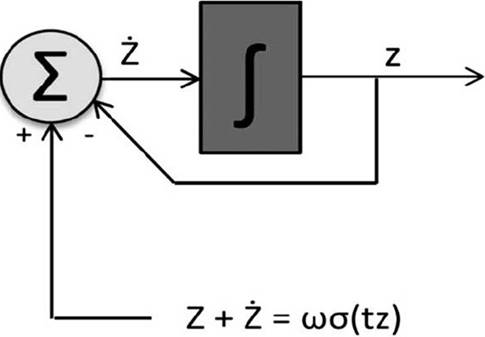Figure 4 Training examples of the multilayer networks in which Z is the output matrix of static layer, Z˙is the derivative with respect to the time of Z , ω is the weights matrix, t is the expected values matrix, and σ is the transfer function.

3. Neuro-spatial variation of properties of rock masses

One of the problems of designing dams is that decisions on very costly expenditure have to be made based on very sparsely sampled information. The volume of samples obtained for characterizing rock masses constitutes only a modest fraction of the volume of material that impacts the design and behaviour of proposed structures.

The engineering properties of rock masses are heterogeneous, with properties varying from location to location. Frequently, however, either due to ease calculation or deficiency of data, geotechnical engineers assume that properties are homogeneous throughout analysed volumes. However, they are aware that this posture can lead to conclusions that significantly differ from real behaviour, and admit that correct knowledge of the spatial distribution of properties promotes safer and more economic designs.

The proposed NN model for determining spatial variation of properties, which is synthesized in Figure 5, is based on the first law of geography "everything is related to everything else, but near things are more related than distant things" (Tobler, 1970). The network is essentially connected with the analysis of a mathematical space (consider that a mathematical space exists whenever a set of observations and quantitative measures of their attributes are available), specifically a geographic one, where the observations correspond to locations in a spatial measurement framework that captures their proximity in the real world.Figure 5 NN topology for spatial variation modelling, the neuro-estimated descriptors are re-integrated as training patterns under the RNN algorithm, RQD is the Rock Quality Designation, %REC is the percentage of recuperation, #FRAC is the number of fractures, °FRAC is the inclination of fractures and MT is the material type.

3.1. Data base description

To demonstrate the convenience of using the neuro-spatial alternative, a 3D characterization of the abutments in a dam site is used. The study area is located in the northern highlands of south-eastern México which are structurally characterized by right-lateral faults in addition to smooth and ample folds of several km. Along the dam area the river develops very close to the Bombana syncline axis, which is segmented toward its south-western flank by the Chicoasén-Malpaso fault, forming complex graben-syncline structures. The local geology of the dam site involves limestones of the Angostura formation (López Ramos, 1969, 1974; Sánchez, 1969; Sánchez et al ., 1978, 1979) in the right abutment, as well as shales, sandstones and conglomerates of the Soyaló formation (González, 1967; López Ramos, 1969, 1974) in the left abutment, both covered by unconsolidated slope deposits and alluvium. The Angostura formation is divided into two units: the inferior unit (Ksa-U1, biogenic limestones) and the superior unit (Ksa-U2, breccias). The Soyaló formation (Tps) consists of shale interbedded with sandstones, conglomerates, calcarenites and calcilutites. (Figure 6a).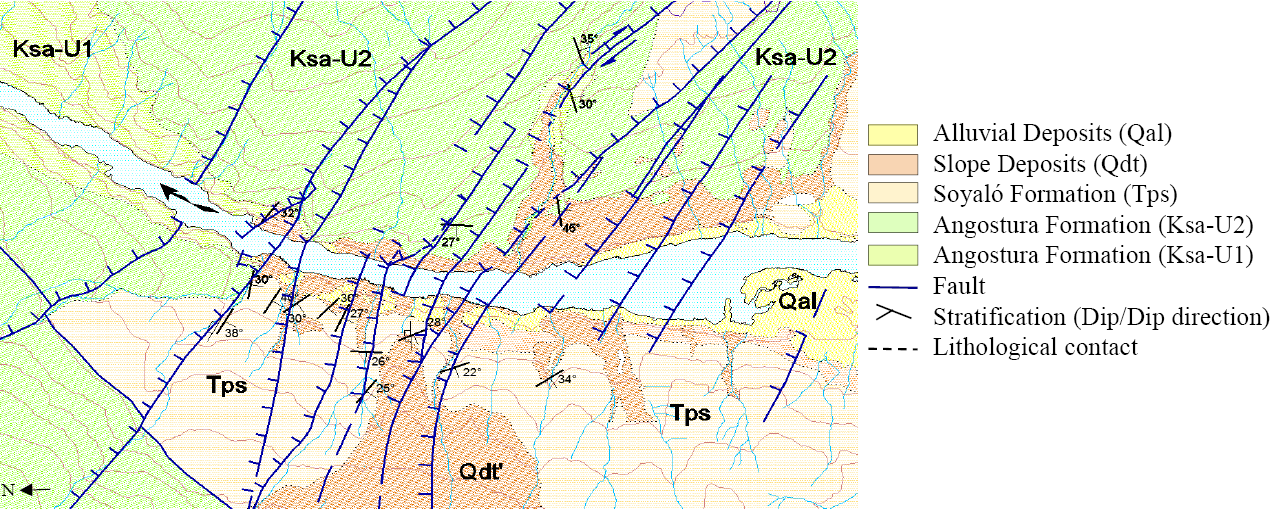Figure 6a Application example: a dam project in the south-eastern of Mexico, geology description.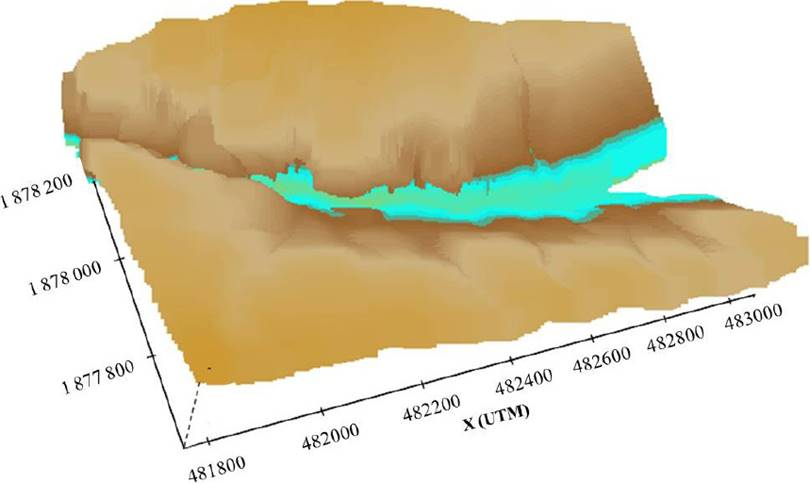Figure 6b Application example: a dam project in the south-eastern of Mexico, abutments 3D view.Figure 6c Application example: a dam project in the south-eastern of Mexico, database, boreholes situation.

In order to evaluate the engineering geological properties of the dam site, 55 boreholes (27 at the right abutment and 28 at the left abutment) were drilled to a maximum depth of 201.2 m. The sum of all the boreholes lengths is 4825 m. In the right abutment, 2309 m were drilled in rock mass (Ksa-U1, Ksa-U2) and 193 m in alluvial deposits. In the left abutment 738 m were drilled in rock mass (Ksa-U1, Ksa-U2), 465 m in alluvial deposits (Qdt, Qal), and 1117 m in Tps material (Table 1).

Overall, this site has been highly affected by tectonic and fault activities. From the drilling results, the designation rock quality (RQD) was evaluated for the right and left abutments (Table 2). The thickness of the weathered zone is variable but bigger in the left abutment.

3.2. Training and validations sets.

From the 55 boreholes, two sets were compiled for modelling each abutment, one for training and one for validation. The training set is composed independently of the validation set. Data in the training set (70 % of the total) are used to adjust the weights on the neural network, while the validation set (the remaining 30 %) is used to minimize overfitting. The validation data are employed to verify that any increase in accuracy over predicting training data actually yields an increase in correctness over cases that have not been shown to the network before (the network is not trained on them). If the accuracy over the training data set grows but not in the validation set then the NN is overfitting and the training should be stopped and a new topology (number of hidden nodes) must be tried. Among data in the validation set, some patterns are selected as test patterns. These data are used only for testing the final solution in order to confirm the actual predictive capacity of the network.

It is important to point out that for collecting data, the determination of the location of each boreholes was randomly distributed over space. Even when the objective was to gather a sample set that represented as much as possible the characteristics of the population in terms of environmental conditions, the cost of experimenting and site accessibility were the main constraints to acquire a good set of measurements. Based on the scarcity of data, to develop 3D models using traditional interpolation methods it is extremely difficult and risky because with such a small number of observations, the approaches could be full of subjective and misleading interpretations.

To train networks under the proposed neuro-spatial model, each sample is represented as a vector with components indicating the position on each axis (X, Y, Z) and the activation level (property value: RQD, %REC, #FRAC, °FRAC and MT) for an output neuron. By assigning to each 3D-inputs (xi, yi, zi) five different output neurons, it is conveniently assumed that the five parameters, interacting, define the quality of rock-mass. Once the NN learns the relations between the position in the space and the rock conditions, the model is able to predict any value of RQD, %REC, #FRAC, °FRAC and MT (as output vector) for any XYZ input.

3.3. Network structure

All of the NNs used were three-layer feed forward networks. As was mentioned, the number of input neurons equalled the number of spatial variables; thus the model had three input neurons xi, yj, zk. The number of output neurons was the number of measured index rock-properties in each abutment (five neurons).

The number of hidden neurons (arranged in one layer) was different for each evaluated NN; the optimum number of nodes was determined though a trial - error approach modified from the method described by Masters (1993). The root mean squares (RMS) were used as criteria to assess networks performance. The RMS measures the difference between the estimated and the prescribed output values for the training set (i.e., how well the results from the network match the observed). The lower the training error is and the higher the test accuracy, the better the network performance. The first trained NN started with 50 hidden neurons that, based on experience, was considered a good starting minimum number for hidden units. When the network was stabilized, that is, the RMS did not diminish in subsequent iterations, the training process was terminated and its efficiency recorded. This hidden layer was then increased by five units, creating a new model, and the training and termination processes were repeated.

The optimum number of hidden neurons is the number at which the network performs well (i.e., with high accuracy and low training error). For the right abutment, this number could be between 560 and 580, and for the left abutment between 650 and 690. Figures 7a and 7b shows the network performance versus the number of hidden neurons for each abutment. In the graphs the number of iterations and the average correlation (sum of the correlations obtained for each output parameter divided by five) generated by each topology is observed.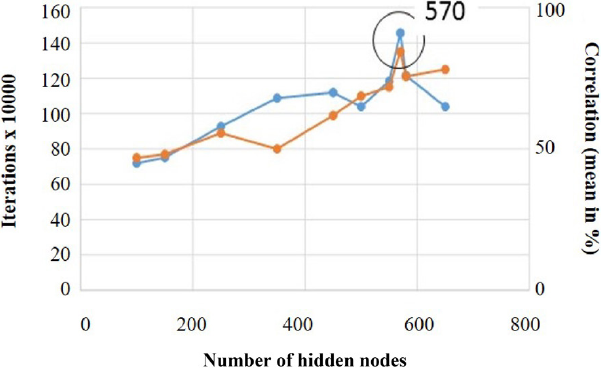Figure 7a Optimum number of hidden neurons a) right abutment.

The final decision among these possible structures was based on the test accuracy for individual properties profiles over the validation set. This is justified because the networks were trained to learn from the training data set. It is possible that with some structures (defined by the number of hidden neurons) the network could be tuned to the training data set too much (overfitting); as a result, the network would not generalize well to a different data set. Thus the selection of the number of hidden neurons should be based more heavily on the test accuracy from the validation data set while still considering the RMS in training.

On the basis of these criteria, the number of hidden neurons in the networks for the right and left abutments was set to 570 and 780, respectively, where test accuracy was highest. Properties profiles (testing data) calculated with the RNN, are shown in Figures 8a and 8b. The remarkable accuracy permits us to label these RNNs as able to reflect the spatial patterns of rock properties. The proposed methodology, them, is effective in the characterisation of both abutments.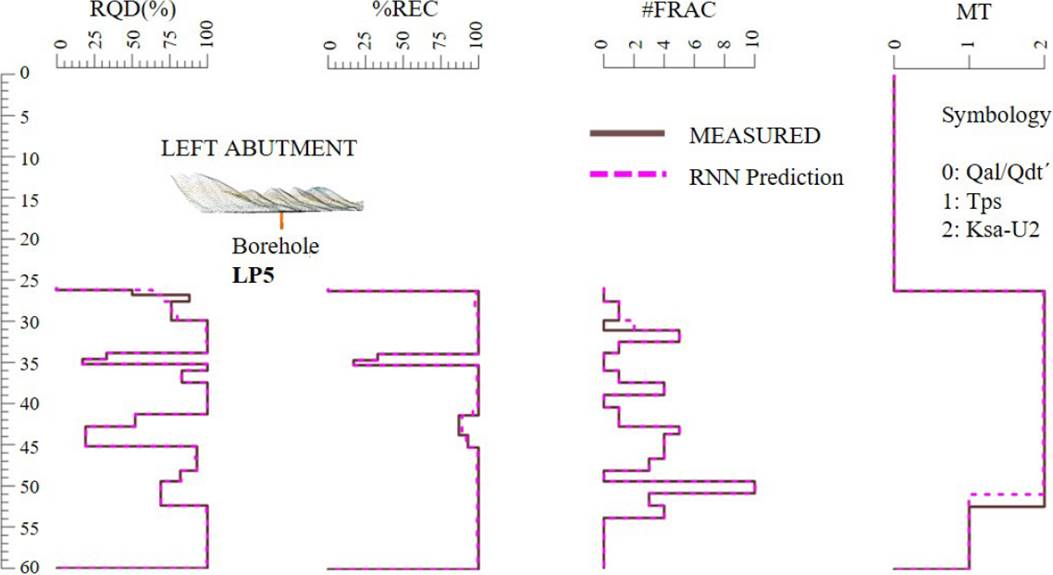Figure 8a Testing the RNN generalization capabilities: continue line in situ measured property, dashed line RNN estimations for "unseen" cases, left abutment.Figure 8b Testing the RNN generalization capabilities: continue line in situ measured property, dashed line RNN estimations for "unseen" cases, right abutment.

3.4. Properties of the abutments: 3D Displays

As previously mentioned, the considered exploration consisted mainly of surface geological surveys, drilling and core recovery, and a few permeability tests in an extension of the Grijalva River of about 1.2 km. Due to economic constraints, restricted accessibility, and delays in construction, a deeper exploration campaign could not be developed, so it was necessary to complete the hydrogeologic model from a minimal database extracted from isolated tests. The importance of developing a 3D definition of properties, as accurate as possible, was amplified by the recognized geological hazards: high-permeability zones and karsticity.

Once the RNNs for both abutments were trained well enough, they were used to populate the vectors of properties for each natural volume. To compute the outputs two matrices of geographic coordinates and depths were presented to the trained networks. Each matrix was constructed with points that fit within the defined borders in the X, Y and Z axes (see the limits depicted in Figures 6b and 6c). The need for a thorough definition of the conditions of the rock led to an optimum spacing in the X and Y axes of 1 m, and 0.5 m in the Z axis. The resulting matrix was 713000 locations on the XY plane and 500 positions in depth for each abutment.

The volumes can be graphically displayed and deeply explored for any X, Y, Z coordinate using the massive 3D environments. Examples of spatial variation of RQD is shown in Figures 9a and 9b and 9c. In Figures 10a and 10b the distribution of #FRAC is presented, while in Figures 11b and MT are shown in Figures 9 to 11. The advantageous ability of the neuronal model to generate detailed descriptions of the volumes of soil and rock is reflected in the example of Figure 9, where the distribution of RQD values can be very useful when selecting the best place to situate the dam project. The traditional 2D conception that designers commonly use for analyzing the information collected on the site is improved using 3D modelling that takes into account the spatial dependency of the whole set of parameters under study. See Figure 10a and 10b.Figure 10a Highly fractured zones, determination of XYZ situations using the RNN calculation, front.Figure 10b Highly fractured zones, determination of XYZ situations using the RNN calculation, plant.Figure 11a Analysis of material distribution, conventional approach.Figure 11b Analysis of material distribution, RNN definition.

To exemplify the neural advantages let's examine one of the most useful parameters in determining the quality of the rock mass the degree of fracturing. This is perhaps one of the most difficult parameters to interpret and include in analyses and models of spatial variation, but the RNNs that are presented in this paper permit us to determine the XYZ situations in which the highest and lowest values of #FRAC can be found (Figure 11). The congruence of the neuro-results was validated using the permeability tests results. An example of immediate application of this type of analysis is shown in Figure 12. When a waterproof screen is being designed, a very common civil work in dam projects, it is necessary to locate the sites of the biggest and smallest leaks. As shown in Figure 12, in a much related way with human interpretation, the neural model helps to define easily and directly these two conditions.Figure 12 Worst and best conditions for construction of waterproof screens, an example of analysis using four parameters.

In short, the 3D definition of index properties permits us to:

• check the hypothesis of conceptual hydrogeological model, previously developed by geologists, and identify areas with very limited information where particular conditions demand further exploration,

• define the most suitable locations of the dam foundation, avoiding areas having possibly the greatest levels of water infiltration, and

• feed functions for estimating the volume of impermeable barriers, acquiring a more precise knowledge about flows of water during different stages of the work.

The NN capacities can be expanded through the integration of more properties; for example results of seismic tomography can be added to better identify important geological faults and their associated properties, and for predicting structural patterns that could have influence on the properties of the rock mass.

It is important to mention that the election of the grid size could have an effect on the displayed variation of properties, particularly when the anisotropy of the rock mass is remarkable. To avoid misconceptions, it is recommended to manage massive sets of 3D properties, by shortening the distance between the neural estimations thereby increasing the effectiveness of the property predictions.

4. Conclusions

From geotechnical and geological perspectives, the proposed methodology, the neural-spatial approximation, is very useful when conventional interpolation methods cannot be applied, for example in sparsely instrumented media. This technique provides valuable data estimation of strata thickness, anomalies, fractured zones and rock structures at locations where no information is available and when is prohibitive to implement statistics or conventional interpolation methods.

The methodology proposed discovers the spatial relations in scarce information from in situ and laboratory tests.

Through a flexible computational organization that the recurrent neural networks provide, the model generates a readable, simple-to-use economical tool for the parallel interpretation of properties of complex natural environments.

References

Csáji, B.C., 2001, Approximation with artificial neural networks: Hungary, Etvs, Lornd University, Faculty of Sciences, 24, 48. [ Links ]

Cybenko, G., 1989, Mathematical problems in neural computing, Signal Processing Scattering and Operator Theory and Numerical Processing, 3, 47-64. [ Links ]

Egmont-Petersen, M., de Ridder, D., Handels, H., 2002, Image processing with neural networks - a review. Pattern Recognition Letters, 35, 2279-2301. [ Links ]

Ghafoori, M., Lashkaripour, G.R., Tarigh-Azali, A., 2011, Investigation of the geological and geotechnical characteristics of Daroongar Dam Northeast Iran: Geotechnical and Geological Engineering, 29, 961-975. [ Links ]

Ghobadi, M.H., Khanlari, G.R., Djalali, H, 2005, Seepage problems in the right abutment of the Shahid Abbaspour Dam, Southern Iran: Engineering Geology, 82, 119-126. [ Links ]

González, A.J., 1967, Exploración Geológica del área Teopizca-Chiapa de Corso, Chiapas: PEMEX Zona Sur: Informe geológico, México, 518, 19-22. [ Links ]

González-Miranda, J.M., 2004, Synchronization and Control of Chaos: An introduction for scientists and engineers, Imperial College Press. ISBN 1-86094-488-4. [ Links ]

Graves, A., 2012, Supervised sequence labelling with recurrent neural networks, Springer-Verlag, Berlin, 385 p. [ Links ]

Hassoun, M., 1995, Fundamentals of Artificial Neural Networks: Cambridge, Massachusetts, MIT Press, London, England, 48 p. [ Links ]

Haykin, S., 1999. Neural networks: a compre-hensive foundation: Upper Saddle River, New Jersey, Prentice Hall, 842 p. [ Links ]

Hinton, G.E., Salakhutdinov, R.R., 2006, Reducing the dimensionality of data with neural networks: Science, 313, 504-507. [ Links ]

Hochreiter, S., Bengio, Y., Frasconi, P., Schmid-huber, J., 2001, Gradient flow in recurrent nets: the difficulty of learning long-term dependencies, in Kremer, S.C., Kolen, J.F., (eds), A Field Guide to Dynamical Recurrent Neural Networks, IEEE Press, USA, 179-203. [ Links ]

Hornik, K., 1991, Approximation capabilities of multilayer feedforward networks: Neural Networks, 4, 251-257. [ Links ]

Jeremias L., Bobby, G, Yung-Cheol B., 2014, An Adaptive Stopping Criterion for Backpropagation Learning in Feedforward Neural Network: International Journal of Multimedia and Ubiquitous Engineering, 9, 149-156. [ Links ]

Kocbay, A., Kilic, R., 2006, Engineering geological assessment of the Obruk dam site Corum, Turkey: Engineering Geology , 87, 141-148. [ Links ]

Lashkaripour, G.R., Ghafoori, M., 2002, The engineering geology of the Tabarak Abad Dam: Engineering Geology Journal, 66, 233-239. [ Links ]

López Ramos, E, 1969, Geología de México: México, D.F., Instituto de Geología, Universidad Nacional Autónoma de México, tomo III, 445p. [ Links ]

López Ramos, E., 1974, Geología general de México: México, D.F., Instituto de Geología, Universsidad Nacional Autónoma de México, 507 p. [ Links ]

Masters T., 1993, Practical Neural Network Recipes in C++: San Diego, California, Academic, 493 p. [ Links ]

McCulloch, W.S., Pitts, W.H., 1943, A Logical Calculus of the Ideas Immanent in Nervous Activity: Bulletin of Mathematical Biophysics, 7, 115-133. [ Links ]

Moler, C., 1984, MathWorks, Massachusetts USA, MathLab, Updated: 2012, available at: <, 1984, MathWorks, Massachusetts USA, MathLab, Updated: 2012, available at: http://www.mathworks.com/ >, consulted on: 2012. [ Links ]

Moreshwar, Z.P., 2013, Applications of Artificial Neural Networks in Civil Engineering, India: University of Pune, Department of Civil Engineering, bachelor thesis, 25p. [ Links ]

Rumelhart, D.E., McClelland, J.L., 1986, On learning the past tense of English verbs, in McClelland, J.L., Rumelhart, D.E., PDP Research Group (eds.), Parallel distributed processing: Explorations in the microstructure of cognition, 2: Psychological and biological models: Cambridge, MA, MIT Press, 216-271. [ Links ]

Sánchez, M. de O., 1969, Proyecto Mesozoico de Chiapas: Chiapas, México: PEMEX Zona Sur, informe geológico, 536, 26-56. [ Links ]

Sánchez, M. de O., Bortolotti, A.C, Angeles, A.F, Espinoza, L.L. Vélez, S.D., 1979, Geología de la Sierra de Chiapas: Boletín de la A.M.G.R, XXXI, 2-6, 67-96. [ Links ]

Sánchez, M. de O., Bortolotti, A.C, Angeles, A.F, Espinoza, L.L., Vélez, S.D., 1978, IX Excursión Geológica, Petróleos Mexicanos. III Excursión Geológica al Sureste de México: Superintendencia General de Distritos de Exploración, Zona Sur, Coatzacoalcos, Veracruz, Reporte técnico, 57p. [ Links ]

Shahin, M.A., Jaksa, M.B., Maier, H.R., 2008, State of the Art of Artificial Neural Networks in Geotechnical Engineering: Electronic Journal of Geotechnical Engineering, ISSN: 1089-3032. [ Links ]

Shahin, M.A., Jaksa, M.B., Maier, H.R., 2009, Recent advances and future challenges for artificial neural systems in geotechnical engineering applications: Journal of Advances in Artificial Neural Systems, 2009, 9p. [ Links ]

Theodoridis, S., Koutroumbas, K., 2009, Pattern Recognition: Theory and Applications, Academic Press, Belgium, 543p. [ Links ]

Tobler, W.R., 1970, A computer model simulation of urban growth in the Detroit region: Economic Geography, 46, Supplement: Proceedings. International Geographical Union, Commission on Quantitative Methods. 234-240. [ Links ]

Uromeihy, A., Farrokhi, R., 2012, Evaluating groutability at the Kamal-Saleh Dam based on Lugeon tests: Bulletin of Engineering Geology and the Environment, 71, 215-219. [ Links ]

Werbos, P.J., 1994, The roots of backpropagation: from ordered derivatives to neural networks and political forecasting: New York, NY, John Wiley & Sons, Inc, 319 p [ Links ]

Received: November 10, 2015; Accepted: April 08, 2016

*Autor para correspondencia: sgab@pumas.iingen.unam.mxThis is an open-access article distributed under the terms of the Creative Commons Attribution License